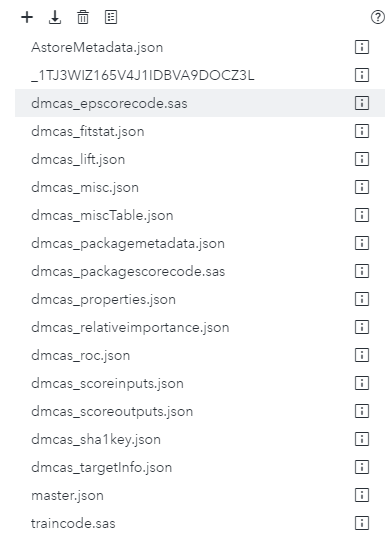## SAS Viya Model Manager Alter Score Code

Hi,

I am trying to add two new variable and basic logic to the score code of a model registered in SAS Viya's (v3.5)  Model Manager (v15.3). I need to publish the model to both CAS and MAS. The logic has worked for the CAS deployment, but not MAS. The model was registered in Model Studio and only the default files are contained in the model project.

I am trying to add these two variable: 'Score' and 'Band'

The logic for the variables are:

``````      Score = round("P_EFT1", 0.01);

if "P_EFT1" > 0.3 then Band = 1;
else if "P_EFT1" > 0.15 then Band = 2;      else Band = 3;``````

The variable 'P_EFT1' is a default output variable from Model Studio.

I have altered the dmcas_epscorecode.sas file, which resulted in the publish to CAS working, but not MAS. I flagged the changes I made with '`/*Added Manually*/`'.

``````data sasep.out;
dcl package score _BE9FNU0BR6ETZUH403ZIEWDLI();
dcl double "P_EFT1" having label n'Predicted: EFT=1';
dcl double "P_EFT0" having label n'Predicted: EFT=0';
dcl nchar(32) "I_EFT" having label n'Into: EFT';
dcl nchar(4) "_WARN_" having label n'Warnings';
dcl double EM_EVENTPROBABILITY;
dcl nchar(8) EM_CLASSIFICATION;
dcl double EM_PROBABILITY;

varlist allvars [_all_];

method init();
_BE9FNU0BR6ETZUH403ZIEWDLI.setvars(allvars);
_BE9FNU0BR6ETZUH403ZIEWDLI.setkey(n'58025805849178A085AE9D34B421C2D28FD2300D');
end;

method post_BE9FNU0BR6ETZUH403ZIEWDLI();
dcl double _P_;

if "P_EFT0" = . then "P_EFT0" = 0.7148;
if "P_EFT1" = . then "P_EFT1" = 0.2852;
if MISSING("I_EFT") then do ;
_P_ = 0.0;
if "P_EFT1" > _P_ then do ;
_P_ = "P_EFT1";
"I_EFT" = '1';
end;
if "P_EFT0" > _P_ then do ;
_P_ = "P_EFT0";
"I_EFT" = '0';
end;
end;
EM_EVENTPROBABILITY = "P_EFT1";
EM_CLASSIFICATION = "I_EFT";
EM_PROBABILITY = MAX("P_EFT1", "P_EFT0");

if "P_EFT1" > 0.3 then Band = 1;/*Added Manually*/
else if "P_EFT1" > 0.15 then Band = 2;/*Added Manually*/

end;

method run();
set SASEP.IN;
_BE9FNU0BR6ETZUH403ZIEWDLI.scoreRecord();
post_BE9FNU0BR6ETZUH403ZIEWDLI();
end;

method term();
end;

enddata;``````

Furthermore, I added the two variables in the 'Variables' tab. The files available in Model Manager are shown below.Can someone please assist with how I can change the code to work for MAS publishing as well? Or maybe even point me to the relevant documentation.

Kind Regards,

Kyle

Discussion stats
• 0 replies
• 396 views
• 0 likes
• 1 in conversation